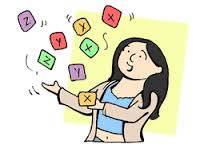# One number is 2 more than 3 times another. Their sum is 22. Find the numbers?

X + (3X + 2) = 22

4X = 20

X = 5

3X + 2 = 17

(5, 17) number set

======

thanked the writer.

The numbers you are looking for are 5 and 17. I worked this out by using the following equation.The first number = x

The other number is  3x+2 in that case, because it is 3 times x plus 2.

x+2+x=22  we add these together knowing that they are equal to 22.
4x+2=22  we then subtract 2 from both sides.
4x=20      Now we divide both sides by 4.
x=5            Which gives us our first number =5.

Okay, so now we need to find the second number:
3x+2= ?  Substitute 5 (the answer from the first part) for x.
3(5)+2=
15+2= 17  Therefore, the 2nd number is 17.

Algebra looks very complicated on the surface, but it gets easier once you understand the methods that are used.

I have included a video that might be helpful (pay no attention to the "for dummies" reference, it's just for beginners).

Once you have the basics, you can work anything out!

thanked the writer.